Op-Amp Experimentation 1: Op-Amp Basics

Op-Amp Experimentation 2: Basic Circuit Math

Op-Amp Experimentation 3: Op-Amp Applications

Op-Amp Experimentation 4: From Ideal to Real

Op-Amp Experimentation 5: Integrator

Op-Amp Experimentation 6: Differentiator

Op Amp Experimentation 7: PID Controller – coming soon

As much as I’d like to, I’m not going to cover basic circuit analysis here. Therefore, this page assumes some familiarity with DC circuit analysis, although I won’t go too deep here. Anyways, this page will cover the basic math involved in analyzing op-amps, which should give us a good preparation for the next page where we cover some applications of op-amps. The main three op-amp configurations will be covered, the non-inverting op-amp, the inverting op-amp, and the differential op-amp. The basic steps involved are the same as any circuit, ie. basic node equations. Just a few things to note, for an ideal op-amp the voltage at the inputs is equal, no current can flow into or out of the inputs, and no current can flow out of the output.

## Example 1: Non-Inverting Op AmpLooking at this, we know that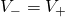and there is no current flowing into or out of the op-amp. Doing a current balance between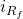and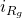yieldswhere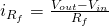and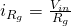.     Substituting these into our current balance yields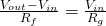.     Solving forresults in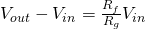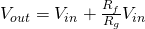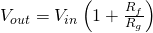Now it is sometimes useful to express the Gain (G) of an op-amp circuit as the ratio of the output to the input.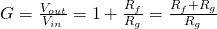Okay, so we went through the theoretical equations. Now lets try some values. Let’s say we are dealing with a small analog sine wave, with an amplitude of 10mV. We want to bump that amplitude up to 1V. So our input is 10mV, output is 1V, our required gain is 1V/10mV = 100. So we would need resistors that follow the ratio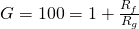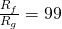This will all get more interesting very soon, I promise. I’ll cover one more example on this page, the inverting amplifier, then the next page will have much more fun things.

## Example 2: Inverting Op-AmpAgain, starting withand knowing that there is no current into or out of the Op Amp, we can start with the current balance at the node betweenand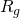.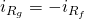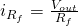Solving for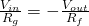Solving for the gain GNotice that this creates an amplified output that is negative. This was probably the trickiest part in going from theoretical circuits to actual circuit designs for me, so I will cover this in more detail later on.

## Example 3: Differential AmplifierHere is a very useful configuration for op-amps. We will analyze this the same exact way as before. First, we setand.

Next we will do a current balance at the node between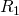and:And a current balance between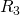and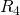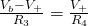.

Substituting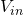in for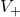and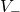and solving for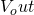Starting with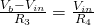we get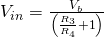Substituting this into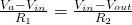results inSimplifying results in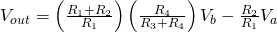Now that doesn’t really look very appetizing, and there is quite a bit more to the math. I’m just going to simplify that by saying if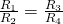then the equation reduces toSo this system just amplifies the difference between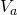and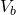. But now that we have a few examples, we can cover some fun tricks you can do with op-amps. Check out the next page for op-amp applications.

Previous page: Op-Amp Experimentation 1: Op-Amp Basics

Op-Amp Experimentation 2: Basic Circuit Math
Tagged on: